Next: Stochastic approximations Up: Posterior approximations Previous: Posterior approximations   Contents

## Model selection

According to the marginalisation principle, the correct way to compare different models in the Bayesian framework is to always use all the models, weighting their results by the respective posterior probabilities. This is a computationally demanding approach and it may be desirable to use only one model, even if it does not lead to optimal results. The rest of the section concentrates on finding suitable criteria for choosing just one best'' model.

Occam's razor is an old scientific principle which states that when trying to explain some phenomenon, the simplest model that can adequately explain it should be chosen. There is no point in choosing an overly complex model when even a much simpler one would do. A very complex model will be able to fit the given data almost perfectly but it will not be able to generalise very well. On the other hand, very simple models will not be able to describe the essential features of the data. One must make a compromise and choose a model with enough but not too much complexity [57,10].

MacKay showed in  how this can be done in the Bayesian framework by evaluating and comparing model evidences. The evidence of a model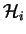is defined as the probability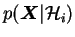of the data given the model. This is just the scaling factor in the denominator of the Bayes theorem in Equation (3.1) and it can be evaluated as shown in Equation (3.2).

True Bayesian comparison of the models would require using Bayes theorem to get the posterior probability of the model as(3.9)

If, however, there is no reason to prefer one model over another, one can choose a uniform prior for all the models. This way the posterior probability of a model is directly proportional to the evidence. There is no need to use a prior on the models to penalise complex ones, the evidence will do that automatically.

Figure 3.2 shows how the model evidence can be used to choose the right model.3.2 In the figure the horizontal axis ranges through all the possible data sets. The curves show the values of evidence for different models and different data sets. As the distributionsare all normalised, the area under each curve is equal.

A simple model like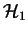can only describe a small range of possible data sets. It gives a high evidence for those but nothing for the rest. A very complex model like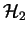can describe a much larger variety of data sets. Therefore it has to spread its predictions more thinly than modeland gives lower evidence for simple data sets. And for a data set like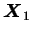lying there in the middle, both the extremes will lose to model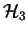which is just good enough for that data and therefore just the model called for by Occam's razor.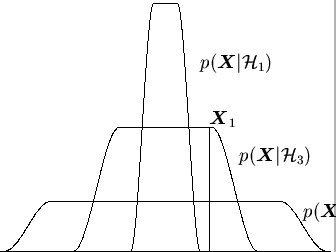After this point the explicit references to the model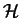in expressions for different probabilities are omitted. This is done purely to simplify the notation. It should be noted that in the Bayesian framework, all the probabilities are conditional to some assumptions because they are always subjective.Next: Stochastic approximations Up: Posterior approximations Previous: Posterior approximations   Contents
Antti Honkela 2001-05-30